User:IssaRice/Computability and logic/Function versus algorithm

If we want to enumerate all of the computable partial functions (say, in order to do some diagonalization argument) the usual way to do this is to enumerate all of the algorithms first, encode the algorithm as an index, and then use this enumeration as an enumeration of the computable partial functions. What this means is that when we refer to some computable partial function as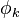$\phi_k$, for example, then actually we know more than just what the function is: we also know that$k$ is an integer that encodes the algorithm that computes$\phi_k$. In contrast, if we just had some computable partial function called$f$, then we know that some algorithm computes$f$, but we have no name for it/a canonical choice for it, so we have to pick some number, say,$n$, so that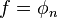$f = \phi_n$.
If we define a function in some exotic way that makes use of something outside our formal definition of computable partial functions, then you won't find the same description in the enumeration, but you will still find the partial function in the enumeration (assuming the partial function can be computed). A silly example that illustrates the point: if we define$f(n)$ to be the number of times Socrates blinked on day$n$ of his life, then this function is computable since we just have to store some finite list of numbers. But you won't be able to find this description of the definition of$f$ in our enumeration. Instead, in the simplest case, you will find a description that looks like a bunch of case statements (if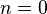$n = 0$ output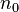$n_0$; else if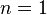$n = 1$ output$n_1$; else if ...). You might also find something that looks like a simulation of the life of Socrates, with hair-counting devices recording the function value on each day.